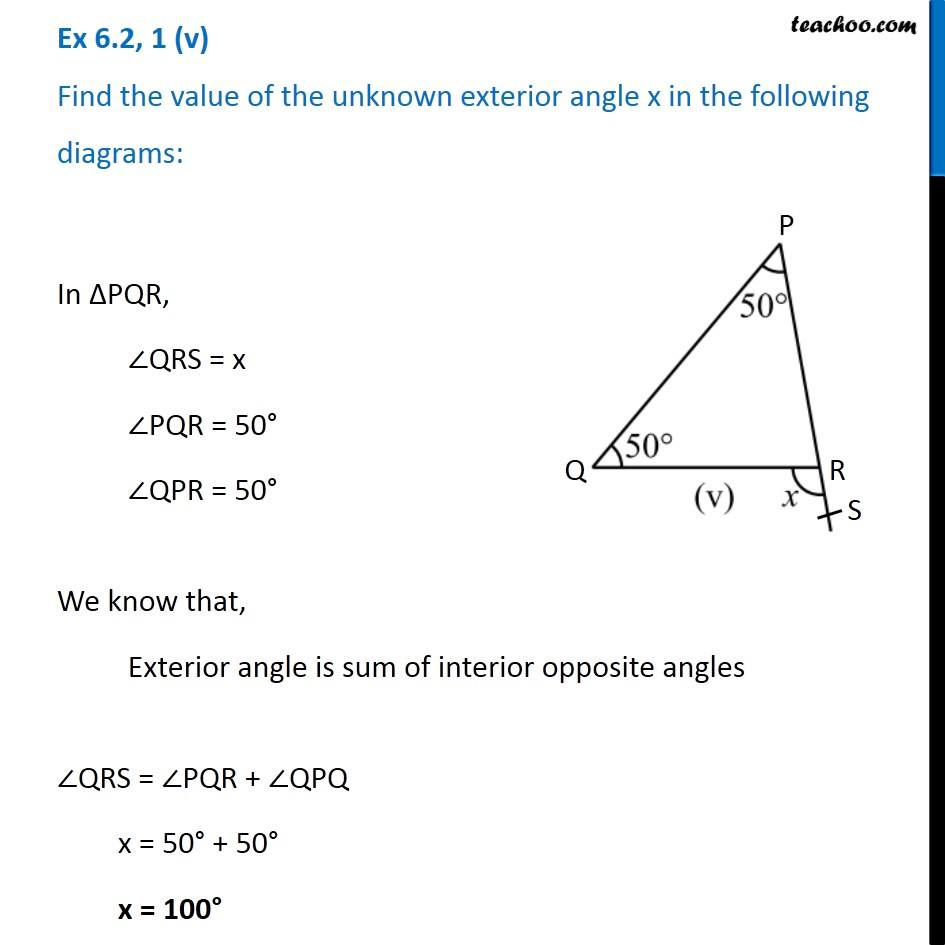Ex 6.2

Chapter 6 Class 7 Triangle and its Properties
Serial order wiseLearn in your speed, with individual attention - Teachoo Maths 1-on-1 Class

### Transcript

Ex 6.2, 1 (v) Find the value of the unknown exterior angle x in the following diagrams:In ∆PQR, ∠QRS = x ∠PQR = 50° ∠QPR = 50° We know that, Exterior angle is sum of interior opposite angles ∠QRS = ∠PQR + ∠QPQ x = 50° + 50° x = 100°